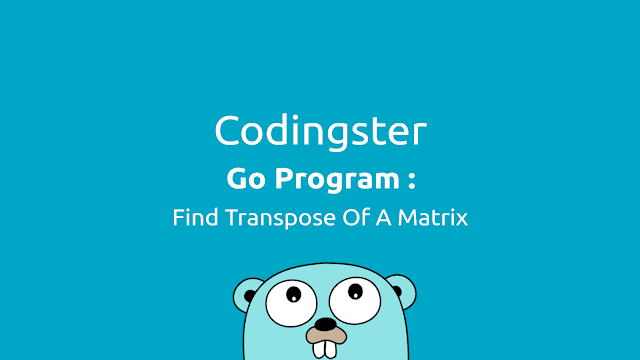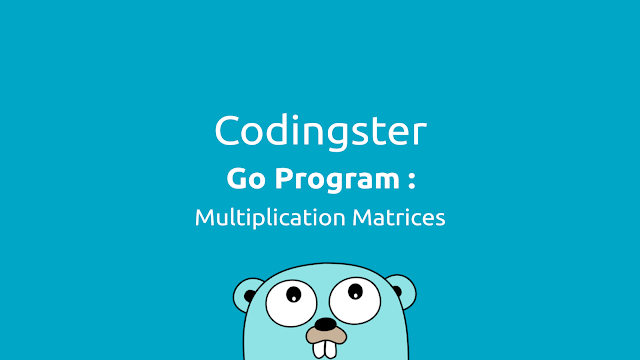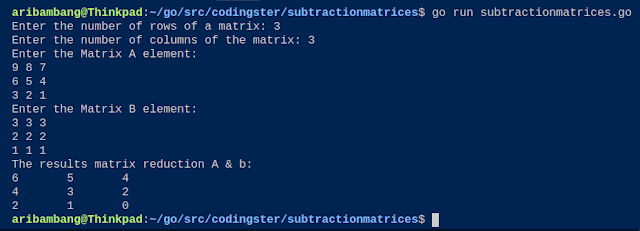-->

## Social Items

Go Program To Check Leap Year - in this post, we will learn how to create a leap year check program in the Go programming language. Leap year is the year where the number of days is the most that occur every four years. The difference in leap year with the normal year is every February has a date up to 29.

## Go Program To Check Leap Year

### Source Code :

``````package main

import "fmt"

func main() {
var year int

fmt.Print("Enter a year: ")
fmt.Scanln(&year)

if year%400 == 0 {
fmt.Println(year, "is a leap year")
} else if year%100 == 0 {
fmt.Println(year, "is not a leap year")
} else if year%4 == 0 {
fmt.Println(year, "is a leap year")
} else {
fmt.Println(year, "is not a leap year")
}
}
``````

Save the source code with the name of leapyear.go, but adjust wrote with a file name that chills and don't forget the extension should .go

### Compile & Run :

Here's how to compile source code manually:
``````
\$ go build leapyear.go
\$ ./leapyear
``````
You can run without having to compile it:
``````
\$ go run leapyear.go
``````

### The Output of Program :Picture of the result check leap year## Go Program To Check Leap Year (Golang)

Go Program To Generate Fibonacci Series (Golang) - in this post we will learn how to create a Fibonacci series program in the Go programming language. Fibonacci is a pattern of numbers obtained from the sum of the two previous numbers in a sequence.

## Go Program To Generate Fibonacci Series (Golang)

### Source Code :

``````package main

import "fmt"

func fibonacci(n int) int {
if n == 0 || n == 1 {
return n
} else {
return (fibonacci(n-1) + fibonacci(n-2))
}
}

func main() {
var n, i, j int
j = 0

fmt.Print("Enter the number of terms: ")
fmt.Scanln(&n)

fmt.Print("Fibonacci series: ")
for i = 1; i <= n; i++ {
fmt.Print(fibonacci(j), ", ")
j++
}
fmt.Println()
}
``````

Save the source code with the name of fibonacci.go, but adjust wrote with a file name that chills and don't forget the extension should .go

### Compile & Run :

Here's how to compile source code manually:
``````
\$ go build fibonacci.go
\$ ./fibonacci
``````
You can run without having to compile it:
``````
\$ go run fibonacci.go
``````

### The Output of Program :Picture of the result Fibonacci series## Go Program To Generate Fibonacci Series (Golang)

Go Program To Find Transpose Of A Matrix (Golang) - In this post, we will learn how to create to find transpose of a matrix program in the Go programming language. Matrix is a collection of numbers arranged in rows (vertical) and columns (horizontal) can also be called two-dimensional arrays. Matrix Transpose produces a matrix by exchanging rows into columns and columns into rows of a matrix.

## Go Program To Find Transpose Of A Matrix (Golang)

### Source Code :

``````package main

import "fmt"

func main() {
var i, j, m, n int
var matrix int
var transpose int

fmt.Print("Enter the number of rows the matrix: ")
fmt.Scanln(&m)
fmt.Print("Enter the number of columns th matrix: ")
fmt.Scanln(&n)

fmt.Println("Enter the matrix elements")
for i = 0; i < m; i++ {
for j = 0; j < n; j++ {
fmt.Scan(&matrix[i][j])
}
}

for i = 0; i < m; i++ {
for j = 0; j < n; j++ {
transpose[j][i] = matrix[i][j]
}
}

fmt.Println("Transpose of matrix: ")
for i = 0; i < n; i++ {
for j = 0; j < m; j++ {
fmt.Print(transpose[i][j], "\t")
}
fmt.Println()
}
}
``````

Save the source code with the name of transpose.go, but adjust wrote with a file name that chills and don't forget the extension should .go

### Compile & Run :

Here's how to compile source code manually:
``````
\$ go build transpose.go
\$ ./transpose
``````
You can run without having to compile it:
``````
\$ go run transpose.go
``````

### The Output of Program :Picture of the result transpose matrix

### Conclusion :

From the results of the program can be run without any error and display the results matrix transpose inputted by the user.## Go Program To Find Transpose Of A Matrix (Golang)

Go Program To Multiplication Two Matrices (Golang) - In this post, we will learn how to create a program calculate the two matrix multiplication in the Go programming language.

Matrix is a collection of numbers arranged in rows (vertical) and columns (horizontal) can also be called two-dimensional arrays. matrix multiplication has the condition that the number of columns of the first matrix is equal to the number of rows of the second matrix.

## Go Program To Multiplication Two Matrices (Golang)

### Source Code:

``````package main

import "fmt"

func main() {

var matrixA int
var matrixB int
var result int
var i, j, k, m, n, p, q, total int

total = 0

fmt.Print("Enter the number of rows the first matrix: ")
fmt.Scanln(&m)
fmt.Print("Enter the number of columns the first matrix: ")
fmt.Scanln(&n)

fmt.Print("Enter the number of rows the second matrix: ")
fmt.Scanln(&p)
fmt.Print("Enter the number of columns the second matrix : ")
fmt.Scanln(&q)

if n != p {
fmt.Println("Error: The matrix cannot be multiplied")
} else {

fmt.Println("Enter the first matrix elements: ")
for i = 0; i < m; i++ {
for j = 0; j < n; j++ {
fmt.Scan(&matrixA[i][j])
}
}

fmt.Println("Enter the second matrix elements: ")
for i = 0; i < p; i++ {
for j = 0; j < q; j++ {
fmt.Scan(&matrixB[i][j])
}
}

for i = 0; i < m; i++ {
for j = 0; j < q; j++ {
for k = 0; k < p; k++ {
total = total + matrixA[i][k]*matrixB[k][j]
}
result[i][j] = total
total = 0
}
}

fmt.Println("Results of matrix multiplication: ")
for i = 0; i < m; i++ {
for j = 0; j < n; j++ {
fmt.Print(result[i][j], "\t")
}
fmt.Print("n")
}
}
}
``````

Save the source code with the name of multiplymatrices.go, but adjust wrote with a file name that chills and don't forget the extension should .go

### Compile & Run :

Here's how to compile source code manually:
``````
\$ go build multiplymatrices.go
\$ ./multiplymatrices
``````
You can run without having to compile it:
``````
\$ go run multiplymatrices.go
``````

### The Output of Program :Picture of result the program

### Conclusion:

From the results of the matrix multiplication program, it can be successfully run without any errors and display the results of the first matrix multiplication and the second matrix with a 3 x 3 order.## Go Program To Multiplication Two Matrices (Golang)

Go Program To Addition Two Matrices - in this post we will learn how to create a program to calculate the addition of two matrices in Go programming language.

Matrix is a set of numbers arranged in rows (vertical) and columns (horizontal) can be referred to as a two-dimensional array. matrix addition have terms that are the order of the matrix must be equal, or in other words, the two matrices must have the number of rows and columns are the same.

### Source Code :

``````
package main

import (
"fmt"
)

func main() {

var i, j, m, n int
var matriksA int
var matriksB int

fmt.Print("Enter the number of rows of a matrix: ")
fmt.Scanln(&m)
fmt.Print("Enter the number of columns of the matrix: ")
fmt.Scanln(&n)

fmt.Println("Enter the Matrix A element: ")
for i = 0; i < m; i++ {
for j = 0; j < n; j++ {
fmt.Scan(&matriksA[i][j])
}
}

fmt.Println("Enter the Matrix B element: ")
for i = 0; i < m; i++ {
for j = 0; j < n; j++ {
fmt.Scan(&matriksB[i][j])
}
}

fmt.Println("The results addition of matrix A & B: ")
for i = 0; i < m; i++ {
for j = 0; j < n; j++ {
fmt.Print(matriksA[i][j]+matriksB[i][j], "\t")
}
fmt.Println()
}
}
``````

Save the source code with the name of additionmatrices.go, but adjust wrote with a file name that chills and don't forget the extension should .go

### Compile & Run :

Here's how to compile source code manually:
``````
\$ go build additionmatrices.go
\$ ./additionmatrices
``````
You can run without having to compile it:
``````
\$ go run additionmatrices.go
``````

### The Output of Program :The Output of Program Addition Two Matrices (Golang)

### Conclusion :

From the results of the matrix addition program can be run without any error and display the results of the addition of matrices A and matrix B.

### Video :## Go Program To Addition Two Matrices (Golang)

Go Program To Subtraction Two Matrices - in this post we will learn how to create a program to calculate the reduction of two matrices in Go programming language.

Matrix is a set of numbers arranged in rows (vertical) and columns (horizontal) can be referred to as a two-dimensional array. matrix reduction have terms that are the order of the matrix must be equal, or in other words, the two matrices must have the number of rows and columns are the same.

### Source Code :

``````
package main

import (
"fmt"
)

func main() {

var i, j, m, n int
var matriksA int
var matriksB int

fmt.Print("Enter the number of rows of a matrix: ")
fmt.Scanln(&m)
fmt.Print("Enter the number of columns of the matrix: ")
fmt.Scanln(&n)

fmt.Println("Enter the Matrix A element: ")
for i = 0; i < m; i++ {
for j = 0; j < n; j++ {
fmt.Scan(&matriksA[i][j])
}
}

fmt.Println("Enter the Matrix B element: ")
for i = 0; i < m; i++ {
for j = 0; j < n; j++ {
fmt.Scan(&matriksB[i][j])
}
}

fmt.Println("The results matrix reduction A & b: ")
for i = 0; i < m; i++ {
for j = 0; j < n; j++ {
fmt.Print(matriksA[i][j]-matriksB[i][j], "\t")
}
fmt.Println()
}
}
``````

Save the source code with the name of subtractionmatrices.go, but adjust wrote with a file name that chills and don't forget the extension should .go

### Compile & Run :

Here's how to compile source code manually:
``````
\$ go build subtractionmatrices.go
\$ ./subtractionmatrices
``````
You can run without having to compile it:
``````
\$ go run subtractionmatrices.go
``````

### The Output of Program :The Output of Program Subtraction Two Matrices (Golang)

### Conclusion :

From the results of the matrix reduction program can be run without any error and display the results of the subtraction of matrices A and matrix B.

### Video :## Go Program To Subtraction Two Matrices (Golang)

Subscribe Our Newsletter